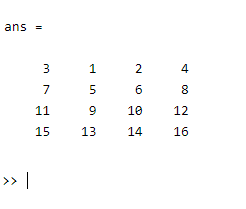Related Articles

# How to Randomly Shuffle Columns in MATLAB in Matrix?

• Last Updated : 27 Aug, 2021

In this article, we are going to discuss the “random shuffling of columns in a Matrix ” with the help of size() and randperm() function. The size() function is used to return the size of each dimension of the specified array “X” or the size of the specified matrix “X”.

## Using Size() and randperm()

1) randperm(): The randperm() function is used for the random permutation of integers.

Syntax:

randperm(n)

randperm(n,k)

Here,

randperm(n) returns a row vector that contains a random permutation of the integers from “1” to “n” without any repetition.

randperm(n,k) returns a row vector that contains “k” a number of unique integers that are selected randomly from 1 to n.

Parameters: This function accepts two parameters.

• n: This is the specified number up to which a random number is going to be generated from “1” without any repetition.
• k: It is the number of unique integers that are selected randomly from 1 to n.

Example:

## Matlab

 `% MATLAB code for calling the randperm()``% to generate a random permutation``% of the integers from 1 to 5``A = randperm(5)`

Output:

```A =
4   2   3   1   5```

Below examples are of the “random shuffling of columns in a Matrix ” which can be done using the combination of the size() and randperm() functions:

2) size: The size() function is used to return the sizes of each dimension of the specified array “X” or the size of the specified matrix “X”.

Syntax:

size(X)

[m,n] = size(X)

size(X,dim)

[d1,d2,d3,…,dn] = size(X)

Here,

size(X) returns the sizes of each dimension of the specified array “X” in a vector d with ndims(X) elements.

[m,n] = size(X) returns the size of the specified matrix “X” in the separate variables m and n.

size(X,dim) returns the size of the dimension of “X” specified by scalar dim.

[d1,d2,d3,…,dn] = size(X) returns the sizes of the first n dimensions of the specified array “X” in separate variables.

Parameters: This function accepts two parameters which are illustrated below:

• X: It is the specified array or matrix or dimension.
• dim: It is the scalar value for the specified dimension “X”

Example 1:

## Matlab

 `% MATLAB code for size() and randpem()``% Specifying a 3*3 matrix``A = [1 2 3``     ``4 5 6``     ``7 8 9];``     ` `% Calling the size() function over``% the above matrix which gives a row vector``% whose elements are the lengths of the``% corresponding dimensions of A``cols = size(A);` `% Calling the randperm() function for the``% random permutation of the above matrix``% over its dimension of 3*3``P = randperm(cols);` `% Getting the column wise randomly shuffled matrix``B = A(:,P)`

Output:

```B =
3   1   2
6   4   5
9   7   8```

Example 2:

## Matlab

 `% MATLAB code for shuffle 4*4 matrix``% using randperm()``% Specifying a 4*4 matrix``A = [1 2 3 4``     ``5 6 7 8``     ``9 10 11 12``     ``13 14 15 16];``     ` `% Calling the randperm() function to``% randomly shuffle the column of matrix A``A(:, randperm(size(A, 2)))`

Output:My Personal Notes arrow_drop_up